# Problems for Chapter 2

2-1. Using the definition of force as the negative of the spatial derivative of the potential energy and the formula for derivative of a polynomial term, describe the motion of a particle under the influence of each of these potential functions:

 U = x U = - x U = y U = - y U = x 2 U = - x 2 U = x 3 U = - x 3 U = 1 / x U = - 1 / x U = 1 / x 2 U = - 1 / x 2 U = x - y U = x 2 + y 2 U = x 2 + 1 / x

In each case, the particle starts out at the point (2,2) and has initial velocity - 1 in the y direction. Be sure to describe its behavior as it crosses any axes, if it does.

Now use the Mathematica notebook "Potential Simulation" to simulate these motions. Discuss the relation between kinetic and potential energy at each place the particle changes direction (ie., the outer limits of oscillation and the point of closest approach for repulsion).

Given the following information:

Proteinv (cm 3 / g)Molecular weightb / b 0
Ribonuclease.70713,6901.066
Myoglobin.74116,9511.105
Lysozyme.70314,3141.21
Immunoglobulin G.739156,0001.513
Myosin.728570,0003.6
Tobacco Mosaic Virus.7340,000,0002.6

Note that "molecular weight" is a "term of art": it is actually a mass (per molecule or per mol; in the table above, the sizes of the numbers indicate that the units are g / mol) and NOT a weight (that is, a force) at all!

The centrifuge rotation rate is 56,050 rpm, each protein has reached about 6.7 cm from the center of rotation, the medium density is 1.01 g / cm 3, and the viscosity of the medium is 0.01003 poise (dyne - sec / cm 2 = g / cm s)

2-2. Compute the mass and volume of each protein.

2-3. Assume the proteins are spheres and compute their radii.

2-4. Use the shape factor in the last column to compute the drag coefficient b for each protein.

2-5. Compute the terminal velocity for each protein in the centrifuge.

2-6. If you can measure to an accuracy of .01 cm, how long do you have to observe the experiment to be able to distinguish the different proteins above? Consider the two proteins which have the closest velocities.

Hint: This is exactly the same problem as the one you did in introductory algebra: if two trains leave Cincinnati for Nashville at the same time, one going 50 mph and the other going 60 mph, when are they 1 mile apart?

2-7. Characterize the dependencies of the terminal velocity on mass, volume and shape.

2-8. For what value of t is the velocity within 1 % of the terminal velocity ? within .01 % ? within .0001 % ? Why the regularity in your results?

2-9. Bovine Ribonuclease has a measured diffusion constant of 13.1 x 10 - 7 cm 2 / sec at 20 C and a specific volume of .707 cm 3 / g. Its molecular weight is 13,690. Compute the drag coefficient b. Assume that the molecule is a sphere and calculate its volume, radius and the corresponding spherical drag coefficient. Find the shape factor b / b 0.

2-10. E. coli DNA ligase has a molecular weight of 74,000, a sedimentation coefficient of 3.81 x 10 - 13 s and a specific volume of .737 cm 3 / g. What is its drag coefficient in a buffer of density 1.01 g / cm 3 ?

2-11. A bacteriophage T7 has a sedimentation coefficient of 453 x 10 - 13 s in water, a measured diffusion constant of 6.03 x 10 - 8 cm 2 / sec at 20 C and a specific volume of .639 cm 3 / g. Calculate its molecular weight.

2-12. Electrophoresis is a sedimentation technique which uses a strong electric field to accelerate charged proteins. Since the charge is proportional to the mass, it allows us to determine the masses of the molecules in the sample. From the Swiss 2-D electrophoresis data base, obtain gel photographs for Plasma and CSF (cerebrospinal fluid). Fit the scale on the left side between 20 and 70 to the following relation:

ln M = a + b x,

where a and b are the fit parameters and x is the displacement along the photo. The scale is in units of Daltons, equivalent to grams per mol. Find the following proteins on the photographs by using their molecular weights and the above equation, with your fitted values of a and b. Be sure to compute a new a and b for each photograph, as the scales are not the same!

ProteinAverage kg / mol
Complement B or C3103
Complement C1S Component89
Alpha-1-B Glycoprotein74
Alpha-1-Antichymotrypsin60
Fibrinogen Gamma-A Chain50
Haptoglobulin-1 Precursor43
Clusterin36
Apolipoprotein30
Immunoglobulin Light Chain25
Plasma Retinol-binding Protein19
Transthyretin14

2-13. If a person's forearm has a cross sectional area of 2.5 cm 2, an effective mass of 3 kg, can normally withstand a maximum compression of 16 x 10 7 Pa (N / m 2) and comes to rest at constant deceleration in 2 ms, what is the maximum speed it could have been going before it hit?

2-14. Estimate the cross sectional area of one of your molars and compute the maximum force it can withstand if its compressive strength is 400 MPa.

2-15. Estimate the height you can safely jump from. Conserve energy to find the speed with which you would hit the ground (the acceleration due to gravity is 9.8 m / s 2).

2-16. Estimate the radius of one of your tibias and using 1700 J / m 2 as the work of fracture, compute the work to fracture it. Why is this number so much smaller than the corresponding one computed in problem # 2-15 ?

2-17. Using the compressional strength from problem # 2-13 and the area from problem # 2-16, find the maximum force which your tibias can withstand.

2-18. If all of your weight was supported by your tibias, what is the minimum time in which you can uniformly decelerate to rest after a fall? Use the velocity from problem # 2-15.

2-19. Estimate the tibia radius and mass for a cat and a mouse. What are the maximum safe falling heights for these two animals? Use the time from problem # 2-18.

2-20. What assumption was made to do problem # 2-19 ?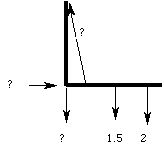Their forearm (including their hand) is 42 cm long and has a mass of 1.5 kg. Their upper arm is 32 cm long. If their biceps muscle is attached to their ulna 3.5 cm from the elbow, what is the tension in their biceps muscle and what are the components of the force exerted on the ulna by the upper arm?

2-22. A person's deltoid muscle is holding their arm horizontally: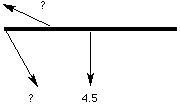It is attached 16 cm from the shoulder, making a 12 degree angle with the horizontal. Their arm is 70 cm long and has a mass of 4.5 kg. What is the magnitude and direction of the force applied by the shoulder, and the tension in the deltoid muscle?

2-23. A person's jaw bone has a horizontal part 7 cm in length and a part at a 50 degree angle from the horizontal, which is 4 cm long: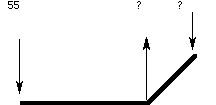The bone pivots at the end of the shorter part. The masseter muscle is attached vertically at the junction of those parts. If the person applies 55 N of force at the front of the jaw bone while chewing, what is the tension in the masseter and the force applied at the pivot?

2-24. A person is holding their lower leg at a 45 degree angle with the vertical: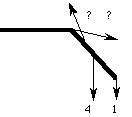Their lower leg has a mass of 4 kg and is 45 cm long, and their foot has a mass of 1 kg. The tendon which holds the leg in place is attached 8 cm from the knee at an angle of 20 degrees to the lower leg. What is the tension in the tendon and the magnitude and direction of the force exerted on the lower leg by the knee?

2-25. A person is standing on tiptoe with their feet at a 30 degree angle from the horizontal: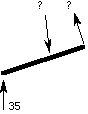Half of their 70 kg mass is supported by the toe. Their foot is 24 cm long and the tibia pushes down at a point 16 cm from the toe. The Achilles tendon acts at a right angle to the foot to pull the person up on the tip of their toes. What is the tension in the Achilles tendon and the magnitude and direction of the force exerted by the tibia?

2-26. A person bends over to pick up a 20 kg mass: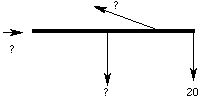Their spine is horizontal. The muscle which supports their upper body makes a 10 degree angle with their spine, and connects 2/3 of the way from the bottom of the spine. What is the tension in that muscle, the compressional force in the spine, and the mass of their upper body?

2-27. A gymnast hangs with arms horizontal from the rings in a move called an "iron cross". One arm is pictured below; if neither arm moves, neither will the gymnast: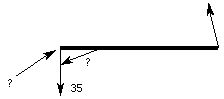Only half of the gymnast's weight is used here; we assume that he is symmetrical. If the vertical component of the tension in the rope is the same as his weight, his mass is 70 kg, his arm is 46 cm long, the muscle responsible for keeping him in place is attached 7 cm from the shoulder at a 30 degree angle from the arm, and the rope hangs at an angle of 5 degrees from the vertical, what is the tension in his muscle and the direction and magnitude of the force on his shoulder joint?

2-28. For each of the skeletal subsystems above, convert the magnitude of the force on the joint to pounds.

2-29. For each of the skeletal subsystems above, given the muscle tensions and weights, why are the forces on the joints in the directions they are?

2-30. Your younger brother has a penchant for practical jokes. He has climbed a tree to a hight of 10 m with a water balloon. Research the compressional strength of the neck and determine the maximum safe mass of the balloon under the following conditions:

• the balloon does not break, and 3/4 of its energy is delivered to the neck in 1 ms.
• the balloon breaks, and 8 % of its energy is delivered to the neck in 1 ms.

2-31. Estimate the length of time it takes to decelerate to rest after a fall. Turn problem 2-18 around: what percentage of your weight is supported by your tibias?

2-32. Assume that the threshold for skull fracture in an automobile accident is 40 mph. If the head has a mass of 5 kg, the area hit is 50 cm 2 and the time to decelerate to rest is inversely proportional to the velocity, find a formula for the deceleration time as a function of velocity.

2-33. It is easier to estimate the braking distance (the distance which the center of mass of an object moves as it decelerates to rest) than the braking time (why?). Compute the maximum height from which you can safely jump to the ground by estimating your braking distance.

2-34. Wile E. Coyote (of Warner Brothers cartoon fame) frequently falls from cliffs while pursuing his intended dinner. Assuming that he falls from cliffs that are approximately 150 m high, and that the pressure he can safely incur (over his .1 m 2 frontal surface area) is 16 MPa, what is the depth of the hole which he must create during braking? This gives us some insight into the compressibility of cartoon dirt.